JMSLTM Numerical Library 7.2.0
com.imsl.math

extends Object
Solves the convex quadratic programming problem subject to equality or inequality constraints.

Class QuadraticProgramming is based on M.J.D. Powell's implementation of the Goldfarb and Idnani dual quadratic programming (QP) algorithm for convex QP problems subject to general linear equality/inequality constraints (Goldfarb and Idnani 1983); i.e., problems of the form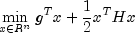subject to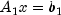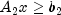given the vectors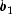,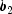, and g, and the matrices H,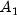, and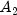. H is required to be positive definite. In this case, a unique x solves the problem or the constraints are inconsistent. If H is not positive definite, a positive definite perturbation of H is used in place of H. For more details, see Powell (1983, 1985).

If a perturbation of H,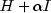, is used in the QP problem, thenalso should be used in the definition of the Lagrange multipliers.

If the constraints are infeasible an exception is thrown. See Example 3 where the exception is caught and printed.

Example 1, Example 2, Example 3
• Field Detail

• EPSILON_SMALL

public static final double EPSILON_SMALL
The smallest relative spacing for doubles.
Constant Field Values
• Method Detail

• getDual

public double[] getDual()
Returns the dual (Lagrange multipliers).
• getOptimalValue

public double getOptimalValue()
Returns the optimal value.
Returns:
a double, the optimal value.
• getSolution

public double[] getSolution()
Returns the solution.
• isNoMoreProgress

public boolean isNoMoreProgress()
Returns true if due to computer rounding error, a change in the variables fail to improve the objective function. Usually the solution is close to optimum.
JMSLTM Numerical Library 7.2.0